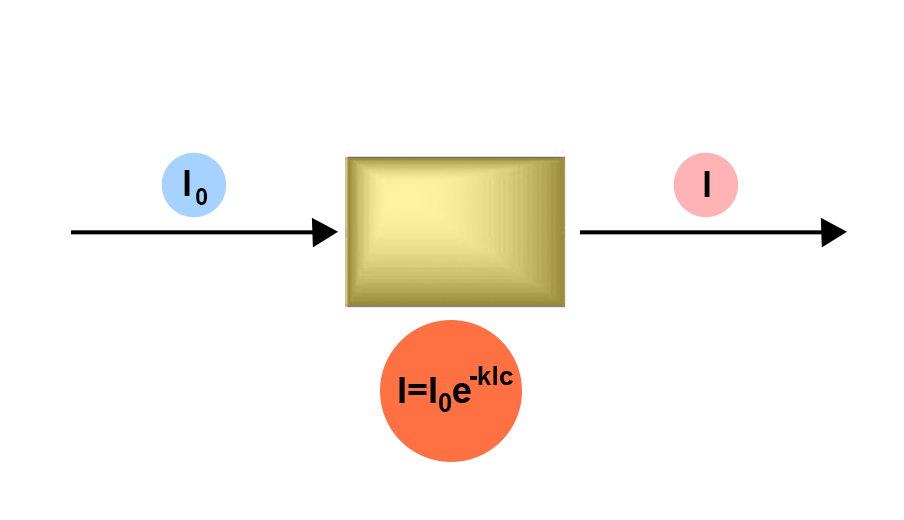### ON WHAT PRINCIPLE?

The Beer-Lambert law:Io: incident beam intensity at wavelength λ
I: intensity of the emergent beam at wavelength λ
l: thickness of the cuvette (1cm)
c: concentration of the solution
K: extinction coefficient: may have several names depending on the unit expression of c
When a light beam of a defined wavelength goes through a solution that has to be analysed, the light intensity that is absorbed by the solution is proportional to the concentration of absorbent substance.
The notion of transmission (T) is used sometimes: T = I/Io x 100
For convenience, the notion of absorbance is defined: A = 1/T being A = εlc

If c is given in g/100 mL: K may be assimilated to E1% (or A11): specific extinction coefficient.
Thus, the specific absorbance is obtained: the absorbance that a solution of a product at a 1% concentration (1g/100mL) would have, if it was measured using a 1 cm cuvette at a given wavelength.

Conditions of validity: the Beer-Lambert law is valid for:
- A given monochromatic light (λmax).
- A low concentration otherwise A=f(c) is not respected anymore.
- A clear solution.
- Stable molecules in solution and under the effect of irradiation.

Next page
Previous page